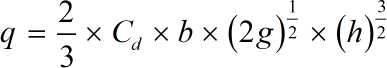Request a Tool

Rectangular Weir Flow Rate Calculator

The Rectangular Weir Flow Rate calculator is used to measure the water flow rate. The flow rate over a weir is a function of the head on the wei.

Flow Rate
0

Formula• g = Gravity Constant value (9.81 m/s²)
• q = Flow rate
• h = Head on the Weir
• b = Width of the Weir
• Cd = Discharge Constant

Defination / Uses

The Rectangular Weir Flow Rate is used to estimate the flow rates of rivers and canals in open channels. The water flow rate in rivers and canals is measured using our powerful online Rectangular Weir Flow Rate Calculator.

How to use rectangular weir flow rate calcultor?

There are only single step.

• Add the values in their corresponding fields.